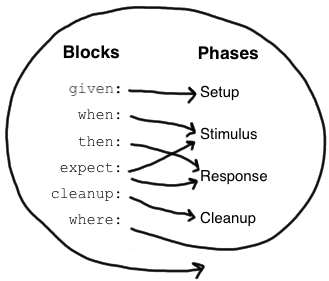# Spock 入门• Setup 初始化
• Stimulus 刺激
• Response 响应
• Cleanup 清理资源

## 块

### Given 块

 ``````1 2 3 `````` ``````given: def stack = new Stack() def elem = "push me" ``````

### When 和 Then 块

when 和 then 块始终一起出现。他们描述了刺激和预期的反应。

 ``````1 2 `````` ``````when: // 刺激 then: // 响应 ``````

 ``````1 2 3 4 5 6 7 `````` ``````when: stack.push(elem) then: !stack.empty stack.size() == 1 stack.peek() == elem ``````

### Expect 块

 ``````1 2 3 4 5 `````` ``````when: def x = Math.max(1, 2) then: x == 2 ``````
 ``````1 2 `````` ``````expect: Math.max(1, 2) == 2 ``````

### Cleanup 块

 ``````1 2 3 4 5 6 7 8 `````` ``````given: def file = new File("/some/path") file.createNewFile() // ... cleanup: file.delete() ``````

### Where 块

 ``````1 2 3 4 5 6 7 8 9 `````` ``````def "computing the maximum of two numbers"() { expect: Math.max(a, b) == c where: a << [5, 3] b << [1, 9] c << [5, 9] } ``````

 ``````1 2 3 4 5 6 7 8 9 `````` ``````def "computing the maximum of two numbers"() { expect: Math.max(a, b) == c where: a | b | c 5 | 1 | 5 3 | 9 | 9 } ``````